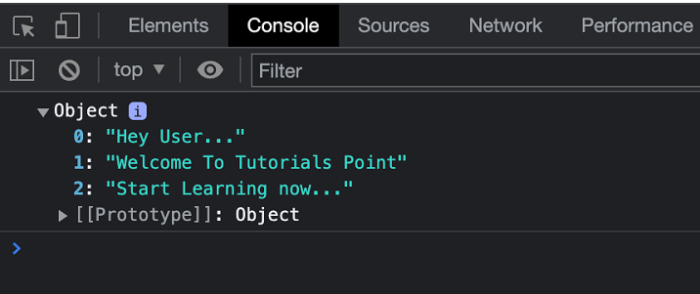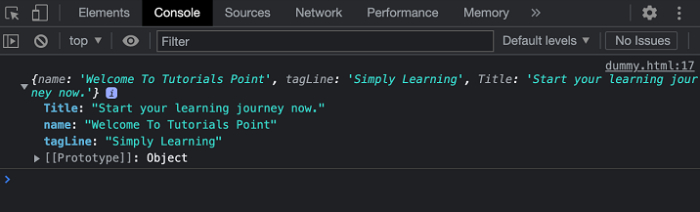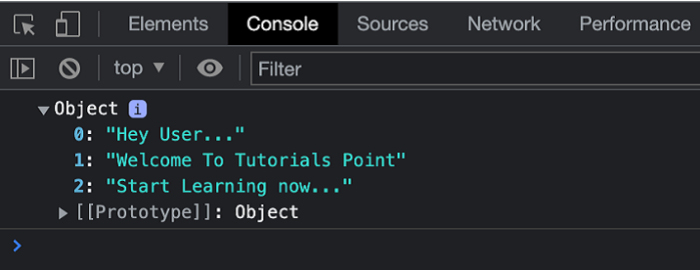# JavaScript: How to Create an Object from Key-Value Pairs

In this article, we are going to explore how to create a JavaScript object from the desired key-value pairs. Let’s understand the steps involved as below−

## Steps:

Step I − We are first going to create an Empty Object.

let object = {}

Step II − Once the object is initialized with the null values we can add the desired {key, value} pairs in the object.

let firstKey = 0;
let firstKeyValue = "TutorialsPoint";
object[firstKey] = firstKeyValue;

Step III − The resultant object is the JSON object that holds all the key-value pairs.

console.log(object);

## Example 1

In this example, we are creating a simple application. In the script part, we have created an empty object and then added some key, value pairs to it. The same is shown on the console page.

# index.html

<!DOCTYPE html>
<html>
<title>Object Using Key-value Pair</title>
<body>
<h1 style="color: green;"> Welcome to Tutorials Point</h1>
<script type="text/javascript">
let object = {};
let firstKey = 0;
let firstKeyValue = "Hey User...";
let secondKey = 1;
let secondKeyValue = "Welcome To Tutorials Point";
let thirdKey = 2;
let thirdKeyValue = "Start Learning now...";
object[firstKey] = firstKeyValue;
object[secondKey] = secondKeyValue;
object[thirdKey] = thirdKeyValue;
console.log(object);
</script>
</body>
</html>

## Output

On successful execution of the above program, the browser will display the following result-

Welcome To Tutorials Point

And in the console, you will find all the results, see the screenshot below −## Example 2

In the example below, we consider that the keys and values are present in an array. We use a for loop over the array, and append data from the arrays into an empty object as key-value pair(s).

# index.html

<!DOCTYPE html>
<html>
<title>Object Using Key-value Pair</title>
<body>
<h1 style="color: green;"> Welcome to Tutorials Point</h1>
<script type="text/javascript">
let object={};
let keys = ["name", "tagLine", "Title"];
let values = ["Welcome To Tutorials Point", "Simply Learning", "Start your learning journey now."];
for (let i = 0; i < keys.length; i++) {
object[keys[i]] = values[i];
}
console.log(object);
</script>
</body>
</html>

## Output

On successful execution of the above program, the browser will display the following result −

Welcome To Tutorials Point

And in the console, you will find all the results, see the screenshot below −## Example 3

In the example below, we use Object.assign() method which is the part of the Object superclass. This method copies all the values and appends those values to the object as key-value pair(s).

# index.html

<!DOCTYPE html>
<html>
<title>Object Using Key-value Pair</title>
<body>
<h1 style="color: green;"> Welcome to Tutorials Point</h1>
<script type="text/javascript">
let object = {};
let firstKey = 0;
let firstKeyValue = "Hey User...";
let secondKey = 1;
let secondKeyValue = "Welcome To Tutorials Point";
let thirdKey = 2;
let thirdKeyValue = "Start Learning now...";
Object.assign(object, { [firstKey]: firstKeyValue });
Object.assign(object, { [secondKey]: secondKeyValue });
Object.assign(object, { [thirdKey]: thirdKeyValue });
console.log(object);
</script>
</body>
</html>

## Output

On successful execution of the above program, the browser will display the following result −

Welcome To Tutorials Point

And in the console, you will find all the results, see the screenshot below −